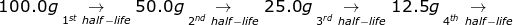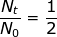# Radioactive Half-Life Formula

Radioactive Half-Life Formula

A radioactive half-life refers to the amount of time it takes for half of the original isotope to decay. For example, if the half-life of a 50.0 gram sample is 3 years, then in 3 years only 25 grams would remain. During the next 3 years, 12.5 grams would remain and so on.Nt = mass of radioactive material at time interval (t)

N0 = mass of the original amount of radioactive material

k = decay constant

t = time interval (t1/2 for the half-life)

Radioactive Half-Life Formula Questions:

1. If the half-life of 100.0 grams of a radioactive isotope is 8 years, how many grams will remain in 32 years?

Answer:

To answer this question, there is no need to solve for the radioactive decay equation. If 32 ÷ 8 = 4, then the material will go through 4 half-lives.6.25g

2. What is the decay constant for a radioactive isotope with a half-life of 22.5 hours?

At first glance, it may seem that not enough information was provided to solve this problem. However, if the half-life value is given for time (t) then the value of(because only half of the original material remains).ln 0.5 = -k(22.5)

-.693 = -k(22.5)

k = 0.0308 hours-1

 Related Links: